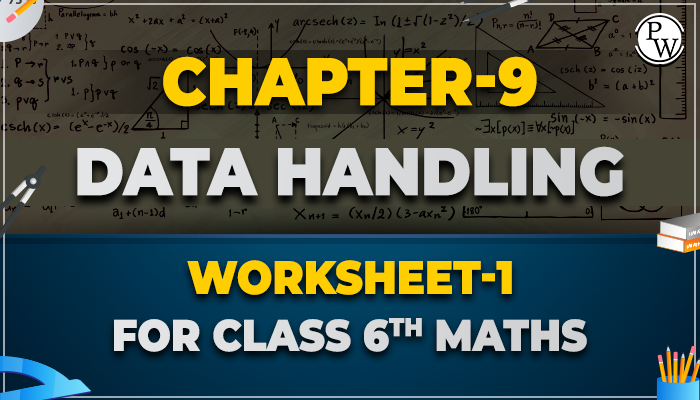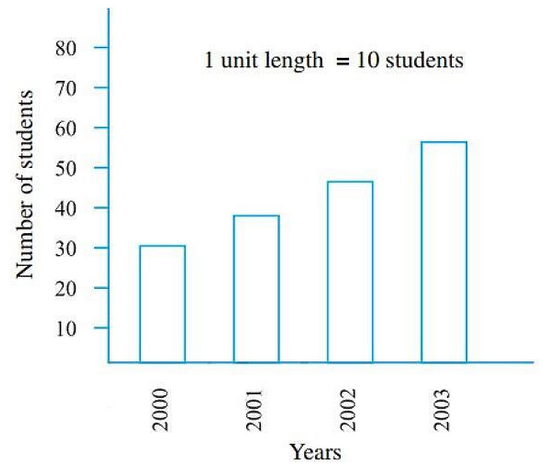# Chapter-9 Data Handling Worksheet-1 for class 6 maths

#### Worksheets for Class 6 Maths

Data handling means collecting data sets and presenting them in a different form. Data is a collection of numbers that represent a specific type of information. The collection of observations that are gathered initially is called raw data. Data can be of any kind. It can be words, numbers, measurements, observations, and descriptions. Data handling is the process of protecting research data collected, archived, or disposed of securely during and after the analysis process.Answer the following question

Q1. In a mathematics test, the following marks were obtained by students:

2, 3, 1, 8, 9, 6, 5, 5, 3, 9, 7, 2, 1, 4, 6, 7, 8, 8, 7, 4, 2, 3,, 7, 8, 9

determine the highest marks.

(a) 8

(b) 9

(c) 7

(d) 10

Ans. Correct option is (b)

In given data, highest marks is 9.

Q2. In a science test of class Vth, the following marks were obtained by 20 student:

3, 6, 7, 6, 9, 7, 10, 7, 8, 6, 3, 8, 7, 6, 7, 9, 7, 8, 6, 7

what is the range of given data?

(a) 7

(b) 6

(c) 8

(d) 5

Ans. Correct option is (a)

The highest marks = 10 The lowest marks = 3

Range of given data = highest marks – lowest marks

= 10 – 3 = 7

Q3. The weight of 25 student of class Xth in kg given as below:

35 , 38 , 40 , 45 , 43 39 , 45.5 , 37, 46, 45, 36, 37, 46, 39, 38, 40, 41, 42, 42, 43, 38, 37, 36, 49, 50.

How many students have 50 or more than weight?

(a) 2

(b) 3

(c) 4

(d) 1

Ans. Correct option is (d)

Only one student has 50 kg weight in given data.

Q4. On the basis of given information answer the following question: in a cricket match score of 7 players are as follow:

32, 95, 1, 70, 29, 75, 19. Lowest and highest score are …….

(a) 1 and 75

(b) 19 and 70

(c) 1 and 70

(d) 1 and 95

Ans. Correct option is (d)

Lowest score is 1, and highest score is 95.

Q5. Read the adjoining bar graph showing the number of students in a particular class of a class and answer the following questionQ6. How many new students are added every year?

(a) 10

(b) 20

(c) 5

(d) 25

Ans. Correct option is (a)

From the bar graph,

The number of student in 2000 year = 30

The number of student in 2001 year = 40 = 30+10

The number of student in 2002 year = 50 = 40+10

The number of student in 2003 year = 60 = 50+10

So, every year added 10 students.

Academic team of Physics Wallah prepared worksheet and questions for chapter Data Handling for all students who are in class 6. Physics Wallah questions for class 6 maths chapter Data Handling consist of carefully selected numerical for class 6 maths and consist of step by step solutions of all questions with answers . Download worksheet-1 of class 6 maths chapter Data Handling and start solving the questions for reference use the solutions . Before solving the worksheet of Physics Wallah it is highly recommend reading the theory of chapter and solve the questions given in your textbook . Do follow NCERT Solutions for class 6 Maths prepared by Physics Wallah.

### Also Check

Below link consist of Class 6 maths Chapter - 9 Data Handling Download your free pdf copy from the below mentioned link of Chapter -  Data Handling.